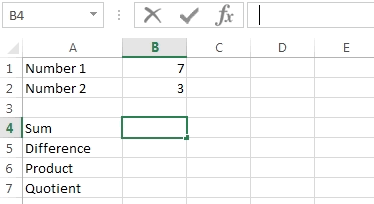### Excel formula

#### Basic Operations | # gomaths.net/4243

Addition, subtraction, multiplication and division.

Addition: `=B1+B2`

Subtraction: `=B1-B2`

Multiplication: `=B1*B2`

Division: `=B1/B2`